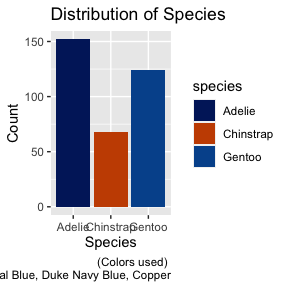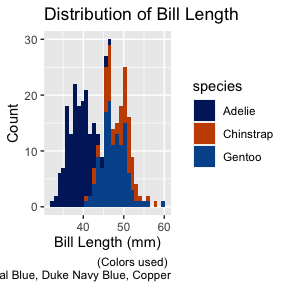# scale_duke_fill_discrete()

This vignette is intended to demonstrate the functionality of scale_duke_fill_discrete(). The function allows users to utilize a discrete color scale of Duke official branding colors on ggplot2 visualizations. It is fully integrated with ggplot2 visualizations.

# Plot Examples

For these visualizations, we will use the penguins dataset from the palmerpenguins package.

library(palmerpenguins)
library(duke)
library(ggplot2)
library(ggmosaic)

## Bar Plot

plot <- ggplot(palmerpenguins::penguins, aes(x = species, fill = species)) +
geom_bar() +
labs(title = "Distribution of Species", caption = "(Colors used) \n Duke Royal Blue, Duke Navy Blue, Copper", x = "Species", y = "Count")

plot +
scale_duke_fill_discrete()## Histogram Plot

plot2 <- ggplot(palmerpenguins::penguins, aes(x = bill_length_mm)) +
geom_histogram(aes(fill = species)) +
labs(title = "Distribution of Bill Length", caption = "(Colors used) \n Duke Royal Blue, Duke Navy Blue, Copper", x = "Bill Length (mm)", y = "Count")

plot2 +
scale_duke_fill_discrete()
#> stat_bin() using bins = 30. Pick better value with binwidth.